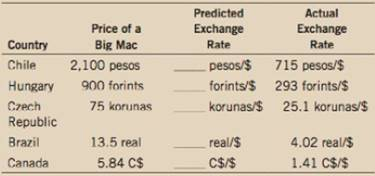Chapter 13, Problem 8PA

Chapter
Section
Textbook Problem

A case study in the chapter analyzed purchasing-power parity for several countries using the price of Big Macs. Here are data for a few more countries:a. For each country, compute the predicted exchange rate of the local currency per U.S. dollar. (Recall that the U.S. price of a Big Mac was $4.93.)b. According to purchasing-power parity, what is the predicted exchange rate between the Hungarian forint and the Canadian dollar? What is the actual exchange rate?c. How well does the theory of purchasing-power parity explain exchange rates? (a): To determine Computation of predicted exchange rate. Explanation If X is the unit of foreign currency per Big Mac and dollar per Big Mac is 3.57, the predicted exchange rate can be found out by dividing 3.57 from unit of foreign currency per Bog Mac (X). This means, it got X3.57 units of foreign currency per dollar. Here the U.S. price of a Big Mac was$4.93, the predicted exchange rate of countries are calculated as follows:

Predicted exchange rate can be calculated by using the following formula:

Predicted exchange rate=PriceIn pesoPriceIn dollar (1)

Substitute the respective values in Equation (1) to calculate the predicted exchange rate in Chili.

Predicted exchange rate=2,1004

(b)

To determine

Computation of predicted exchange rate.

(c)

To determine

Relevance of exchange rate.

Still sussing out bartleby?

Check out a sample textbook solution.

See a sample solution

The Solution to Your Study Problems

Bartleby provides explanations to thousands of textbook problems written by our experts, many with advanced degrees!

Get Started

Define the term budget. How are budgets used in planning?

Managerial Accounting: The Cornerstone of Business Decision-Making

Define the term product

MKTG 12:STUDENT ED.-TEXT

Discuss the following statement: All else equal, firms with relatively stable sales are able to carry relativel...

Fundamentals of Financial Management, Concise Edition (with Thomson ONE - Business School Edition, 1 term (6 months) Printed Access Card) (MindTap Course List)